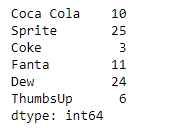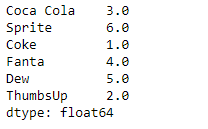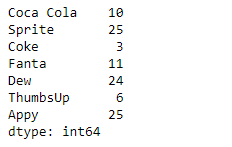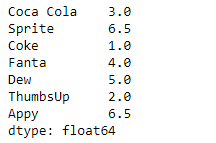# Python | Pandas Series.rank()

• Last Updated : 11 Feb, 2019

Pandas series is a One-dimensional ndarray with axis labels. The labels need not be unique but must be a hashable type. The object supports both integer- and label-based indexing and provides a host of methods for performing operations involving the index.

Pandas` Series.rank()` function compute numerical data ranks (1 through n) along axis. Equal values are assigned a rank that is the average of the ranks of those values.

Attention geek! Strengthen your foundations with the Python Programming Foundation Course and learn the basics.

To begin with, your interview preparations Enhance your Data Structures concepts with the Python DS Course. And to begin with your Machine Learning Journey, join the Machine Learning - Basic Level Course

Syntax: Series.rank(axis=0, method=’average’, numeric_only=None, na_option=’keep’, ascending=True, pct=False)

Parameter :
axis : index to direct ranking
method : {‘average’, ‘min’, ‘max’, ‘first’, ‘dense’}
numeric_only : Include only float, int, boolean data. Valid only for DataFrame or Panel objects
na_option : {‘keep’, ‘top’, ‘bottom’}
ascending : False for ranks by high (1) to low (N)
pct : Computes percentage rank of data

Returns : ranks : same type as caller

Example #1: Use `Series.rank()` function to rank the underlying data of the given Series object.

 `# importing pandas as pd``import` `pandas as pd`` ` `# Creating the Series``sr ``=` `pd.Series([``10``, ``25``, ``3``, ``11``, ``24``, ``6``])`` ` `# Create the Index``index_ ``=` `[``'Coca Cola'``, ``'Sprite'``, ``'Coke'``, ``'Fanta'``, ``'Dew'``, ``'ThumbsUp'``]`` ` `# set the index``sr.index ``=` `index_`` ` `# Print the series``print``(sr)`

Output :Now we will use `Series.rank()` function to return the rank of the underlying data of the given Series object.

 `# assign rank``result ``=` `sr.rank()`` ` `# Print the result``print``(result)`

Output :As we can see in the output, the `Series.rank()` function has assigned rank to each element of the given Series object.

Example #2: Use `Series.rank()` function to rank the underlying data of the given Series object. The given data also contains some equal values.

 `# importing pandas as pd``import` `pandas as pd`` ` `# Creating the Series``sr ``=` `pd.Series([``10``, ``25``, ``3``, ``11``, ``24``, ``6``, ``25``])`` ` `# Create the Index``index_ ``=` `[``'Coca Cola'``, ``'Sprite'``, ``'Coke'``, ``'Fanta'``, ``'Dew'``, ``'ThumbsUp'``, ``'Appy'``]`` ` `# set the index``sr.index ``=` `index_`` ` `# Print the series``print``(sr)`

Output :Now we will use `Series.rank()` function to return the rank of the underlying data of the given Series object.

 `# assign rank``result ``=` `sr.rank()`` ` `# Print the result``print``(result)`

Output :As we can see in the output, the `Series.rank()` function has assigned rank to each element of the given Series object. Notice equal values has been assigned a rank which is the average of their ranks.

My Personal Notes arrow_drop_up